# Catch up on the latest AI articles# Generative Model For Real-world Time Series Data

3 main points
✔️ Propose a generative model for time series data
✔️ The model does not require fine-tuning of AE-GAN
✔️ Even in the presence of missing data, data generation is possible through observation embedding and decision and generation algorithms in the decoder

written by Hengzhi PeiKan RenYuqing YangChang LiuTao QinDongsheng Li
(Submitted on 16 Nov 2021)
Accepted in 21th IEEE International Conference on Data Mining (ICDM 2021)
Subjects: Machine Learning (cs.LG); Artificial Intelligence (cs.AI)code：The images used in this article are from the paper, the introductory slides, or were created based on them.

## first of all

Time series data recorded by sensors will be ubiquitous in healthcare, agriculture, manufacturing, and many other sectors. However, much of this data is sensitive and may pose privacy and accessibility issues when used, for example, in inpatient records. Recently, generating synthetic data for applications such as follow-up machine learning tasks has become one of the promising solutions. While there are no theoretical guarantees, recent work has demonstrated that the generated data is resilient to membership inference attacks, patient re-identification, etc. More importantly, generated data is often more useful than anonymized/perturbed methods.

Generating realistic time series data is a challenging problem. A good generative model needs to capture not only the multidimensional distribution at each time point but also the temporal dynamics over time. In addition, synthetic time series need to reflect corresponding global features, e.g., static features such as age, as well as labels of interest such as mortality in clinical data. With the recent success of Generative Adversarial Networks (GANs) and their variants, it is natural to extend the GAN framework for time series data generation by applying Recurrent Neural Networks (RNNs). Generators and Discriminators, Following this paradigm, several works have been proposed to solve the problem of generating time series data. However, these works are usually targeted at the generation of simple and properly formatted time series data and may not apply to the generation of real-time series data, as shown in empirical studies.

Incomplete real-world time series data pose new challenges to data generation algorithms. 1) Long sequences of variable length: real-world time series data are long, variable in length, and some cases can be significant. For example, survival analysis. However, many existing methods have only been evaluated on short fixed-length time series, and their performance on long variable-length time series remains unexplored. 2) Missing values: Missing values are very common in real-time series data. An example of clinical data for mortality prediction is shown in Fig. 1. These missing values can be informative. For example, missing values in clinical data may reflect the patient's situation and the physician's decision. And more and more research is focused on exploiting missing patterns to improve predictive performance. However, to the best of our knowledge, none of the existing studies have examined the use of informative missing values to generate time series.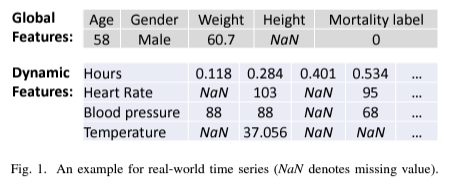In this paper, we propose a novel generative framework, Real-World Time Series Generative Adversarial Network (RTSGAN), to address the aforementioned challenges. RTSGAN consists of two main components: 1) an encoder-decoder module that encodes each time series instance into a fixed-dimensional latent vector and learns to reconstruct the entire time series from the latent vector via an autoencoder. 2) The generator module: WassersteinGAN (WGAN), which is trained to generate vectors in the same latent space as the autoencoders above. Using the generator and decoder, RTSGAN can generate real-world time-series data that respect the original feature distribution and temporal dynamics. To better address the problem of beneficial missingness, RTSGAN is extended to RTSGAN-M. Observation embedding is proposed to enrich the information at each time step, and a new decision and generation decoder is also proposed to first determine the time and missing patterns. In the next step, the corresponding function values are generated based on both local and global dependencies. An empirical study on four real-world time series datasets shows that the synthetic data generated by the proposed framework not only looks more "realistic", but is also more useful for downstream machine learning tasks in "training the synthesis, testing the real world".

The main contributions of this paper are as follows

-To address the challenges posed by real-world time series data, we propose a new time series data generation framework named RTSGAN.

-To the best of our knowledge, this is the first study to investigate the problem of generating time series with missing values, and RTSGAN-M observation embedding and a new decision and generation decoder are proposed to achieve better generation performance.

-The detailed experiments were conducted on four real-world datasets containing both complete fixed-length and incomplete variable-length time series, and RTSGAN was used to perform the downstream classification and prediction tasks. and outperforms the SOTA method in terms of synthetic data usage in

In general, each instance of time series data is composed of two main types of feature values. Dynamic feature values (which change over time, such as the heart rate of a single patient) and global feature values (which include static features, such as age, and global properties of the observed sequence, e.g., our labels of interest). Feature values for both dynamic and global feature values are continuous values or categorical variables. We denote one instance of the time series training set D as (X, y). Here, $X = ( x1, . , xl ) ∈ R^{l×d}_x$ represents a dx-dimensional multivariate time series containing l observables. y ∈ $R^{d_y}$ represents a global feature of the time series. In practice, time-series data may be incomplete. That is, missing values in the time series data may occur in general for both dynamic and global feature values, as shown in Fig. 1. To formulate this problem, we denote each instance as $(X, y, M^{(x)}, m^{(y)})$. A mask matrix M (x) ∈ Rl×K is introduced to represent the missing values of the K dynamic features. Here, $M^{(x)}_{i, j} = 1$ if the jth feature is observed in the i-th observation, and 0 otherwise. Similarly, $M(y) represents the missing global feature value. The goal of this work is to use the training set D to learn data distributions and generate a synthetic dataset$ \hat{D} $with a realistic appearance and high usefulness. Downstream machine learning tasks such as classification and sequence prediction can be performed on the synthetic dataset$ \hat{D} $, and the resulting downstream models can perform similarly compared to models trained on D. ## RTSGAN method The architecture of RTSGAN is illustrated in Fig. 2 and consists of the following two key modules Encoder-Decoder Module The time-series data is first learned by the autoencoder and encoded into a fixed-length latent space. The dimension is an invariant of the sequence length. Generation Module After training the encoder-decoder module, the WGAN ( WassersteinGAN) framework is applied to perform generative modeling of the latent space. The generator outputs a synthetic latent representation.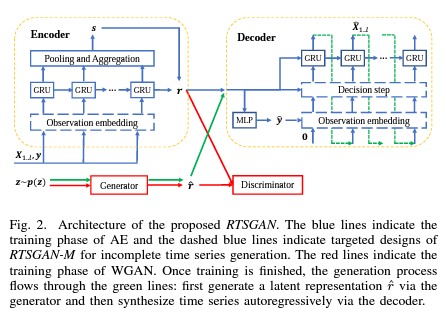To generate the time series, we only need to feed the synthetic latent vector from the generator to the decoder. Previous GAN-based methods required the identification of a vector sequence in either feature value space or latent space. In contrast, RTSGAN only identifies a single latent vector. As the time series data becomes more complex, RTSGAN makes it much easier to understand the original data structure. ## A. RTSGAN with full-time series Encoder-Decoder Module This module consists of an encoder that encodes the input sequence into a latent vector and a decoder that reconstructs the input sequence from the latent vector. Before input to the autoencoder, all functions are converted to [0, 1], min-max scaling is used for continuous functions, and one-hot encoding is used for categorical functions. encoder Unlike the TimeGAN encoder, which encodes each time series into a sequence of latent vectors, the encoder here aims to encode each time series into a compact representation with dimensions invariant to the length of the sequence. First, at each step, the global feature value y is concatenated to the dynamic feature value xi as ei = [ xi, y], which is then fed into an N-layer gated regression unit (GRU) with hidden dimension dAE, and Step i in each GRU layer n Get the hidden state$ h^n_i $for each.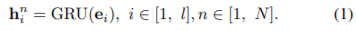To better capture the temporal dynamics and global properties of the time series, the hidden state from the last layer of the GRU$ h^N_i $to further apply the pooling operation to enhance the representation is as follows.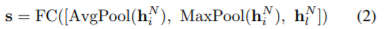Here, FC denotes the fully coupled layer that aggregates the pooling results into the space$ R^{dAE} $, where LeakyReLU is used as the activation function. Next, the global information s and the last hidden state are concatenated to obtain the latent representation r ∈ R. decoder The decoder aims to reconstruct the entire time series from the latent representation r. This involves two steps. (1) First, through a fully connected layer, the global feature value$ \hat{y} $is reconstructed. 2) Next, reconstruct the dynamic feature value$ h^n_l $through the GRU. The global feature value$ \hat{y} $is reconstructed as follows.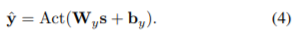In the Act function, softmax is used for categorical feature values and continuous feature values, and sigmoid for continuous feature values. Next, we reconstruct the dynamic feature values. The decoder for dynamic feature values is another N-layer GRU with a hidden dimension dAE that takes$ h^n_l $as the initial hidden state$hˆn_0 $. The reconstruction process is. each p(xi | x1..i-1, y) as follows. It is an autoregressive process that aims to model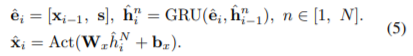The initial input at the start of the autoregressive process is$ \hat{e}ˆ1 $= [0, s]. To handle variable-length time series, we include the sequence length l as one of the global feature values. Thus, after the global feature is reconstructed, we can precisely control the reconstruction of the dynamic feature values with$ \hat{l} $. Autoregressive recurrent networks can be trained using supervised forcing, which always uses the ground-truth data xi-1 as input for the next step, or by sampling the previous prediction$ \hat{x}_{i-1} $and the ground-truth xi-1. The overall loss function is a linear combination of the global feature values and the reconstruction loss of the dynamics feature values.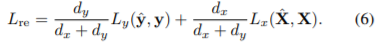Cross entropy (CE) loss and mean squared error (MSE) loss are used for categorical and continuous feature values, respectively. Generation Module As shown above, since both the global and dynamic feature values are encoded in the same latent space, r naturally contains different relations within the time series, and the autoregressive decoder itself maintains the temporal dynamics of the time series. Thus, instead of synthesizing a representation in the latent space and then autoregressively decoding it to produce a synthetic output directly from the feature space, the entire time series can be generated. Since the dimension of the latent space is invariant to the length l of the sequence, it is much easier for the generation module to synthesize the latent representation. Here, we employ an improved version of WGAN. The goal of the WGAN generator is to minimize the 1-Wasserstein distance W (Pr, Pg) between the actual and synthetic data distributions with the help of an iteratively trained 1-Lipschitz discriminator. The optimization objective of WGAN is defined as follows.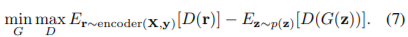Here, G and D denote the generator and the 1-Lipschitz discriminator, respectively. In practice, we use a multilayer perceptron (MLP) with layer normalization for G and three fully coupled layers for D. LeakyReLU is used as the activation function for both G and D. After training the WGAN, we can generate the time series data as follows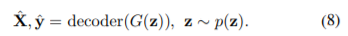Conventional AE-GAN-based generative models require tuning of decoder parameters during GAN training to discriminate real and synthetic data in the feature value space, but in the RTSGAN method, discriminative data are in the latent space and good generative performance can be obtained without fine-tuning features. Therefore, the encoder-decoder module and the generation module are trained separately. ## B. RTSGAN with incomplete time series In this section, we extend RTSGAN to generate incomplete time series. The main idea is to generate both missing vectors and feature vectors at each time step and mask the corresponding feature values according to the generated missing vectors. A simple way to achieve this is to treat the missing information of each feature as an additional binary feature and generate it as a complete-time series. However, a high percentage of missing values in the actual time series data can cause a catastrophic collapse in traditional GAN training. This is because the direct identification of incomplete time series may not provide a useful signal to the generator. For this purpose, a variant of RTSGAN named RTSGAN-M has been proposed. In this variant, the same AE-GAN framework is used, but two techniques are applied in the encoder-decoder module to improve the performance of generating time series data with missing values: 1) Observation embedding: this allows enriching the information in each observation. 2) Decision and generation decoder: it first determines the time and missing pattern of the next observation and then generates the corresponding feature values based on both local and global dependencies. The generation module has not been modified in RTSGAN-M. embedding Before feeding the time series into the encoder-decoder module, an observation embedding layer is added to enhance the representation of the time series at each step. First, some features that are not measured frequently may be stable, so it is natural to consider the last valid observation at each step. Second, the time point ti of each observation should be emphasized, especially for irregular sampling time series. This is because the time point of observation is often related to the missing rate, and the time interval often reflects the influence of previous observations. Despite its ability to capture sequence order, the RNN may not be sensitive enough to capture information from time intervals. Similar to the positional encoding used in Transformer, an embedding layer of points in time is required to fully exploit the temporal information. Therefore, we use the feature values, missing patterns, and time points to construct the observation embedding as follows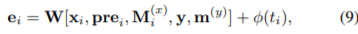where prei denotes the last observation of the dynamic feature before the i-th observation. φ(ti) is the learnable time representation. In doing so, the encoder-decoder module can easily understand the relationships between points in time, missing values, and observed values. The parameters of the overall observation embedding are shared between the encoder and decoder. Determine and generate decoders In many real-world applications, useful missing values can be related to sampling decisions. For example, a physician can decide which dynamic feature to measure next depending on the patient's situation. Thus, by the following conditional distribution$ p(x_i, M^{(x)}_i | info_{i-1}) It is more reasonable to model $.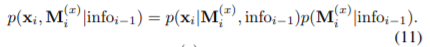where$ info_i := {x_{1..i}, M^{(x)_{1..i}, y, m^{(y)}} $means all information up to the i-th observation.$ p(x_i|M^{(x)}_i, info_{i-1}) $is a model of the dynamic feature value distribution conditioned on other information.$ p(M^{(x)}_i|infor_{i-1}) $is a model of the distribution of missing data conditioned on previous information. Following the ideas above, we split dynamic reconstruction into two steps. Decision and Generation. The decision step consists of the Ndec layer GRU, which generates the point in time and masks for the next observation as follows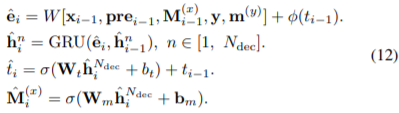Using specific thresholds, we can determine the feature values generated by the ith observation according to$ \hat{M}(x)_i $. After deciding on$ \hat{t}_i $and$ \ hat{M}(x)_i $, we need to take a generative step and model & p(x_i|M^{(x)}_i, infor_{i-1})$. Now we introduce the concept of time delay (the time interval between two consecutive valid observations of a feature value). The time delay δ can be calculated as follows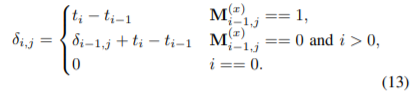Previous work has shown that modifiable time delays are important and that if some features are missing for a long period, the effect of past observations should be reduced. Following this idea, we also introduce trainable decay as follows.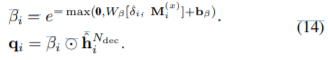qi is the local information estimated at the next point in time $\hat{t}_i$.

This is used together with the global information s to generate dynamic feature values in the (N - Ndec ) layer GRU.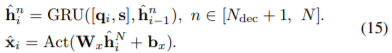s comes from the pooling operation of the entire time series, which is later monitored by the global feature. qi and s can be used to explore both local and global dependencies simultaneously to reconstruct feature values.

Missing value processing and loss functions

In this section, we present a loss function for training the encoder-decoder module under incomplete time series data sets. It consists of the loss of feature value reconstructions and the loss of missing reconstructions. For the reconstruction of incomplete time series of features, only the loss of valid observations is computed. In the case of missing reconstructions, we use binary cross-entropy (BCE) as the loss function. Since missing values of some features may occur frequently, we should try to maintain observations of dynamic features that are seldom observed in each time series. Therefore, we set the rescaling weights wi and j for dynamic features xi and j according to the overall missing rate ρj of the jth dynamic feature as follows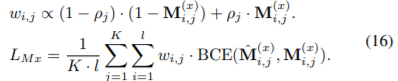The new loss function for dynamic feature values looks like this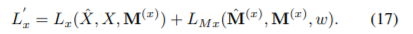The loss of the global feature value $\prime{L}_y$ is similarly into two components. which can be decomposed. The overall loss function can then be derived as in Equation 6.

## experiment

We evaluate RTSGAN in terms of 1) realistic data 2) high usefulness.

A. Full fixed-length time-series data

The datasets used in the evaluation are Google's stock price and UCI Appliance's energy consumption forecast data. From each data set, we extract 3,773 time series of length 24 from the stock price and 19,711 from the energy forecast. The methods we compare are COT-GAN, TimeGAN, RCGAN, C-RNN-GAN, WaveNet, and WaveGAN. Discrimination score and prediction score are used as evaluation metrics, and t-SNE and PCA are used for qualitative evaluation to evaluate how close the real data is to the generated data.

The results are shown in TABLE 1, Fig. 3, where we can see that RTSGAN scores the best and that the generated data (blue) best reproduces the original distribution (red) for RTSGAN as well as for t-SNE and PCA.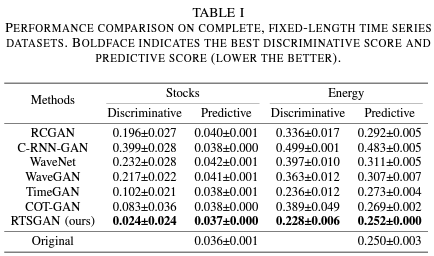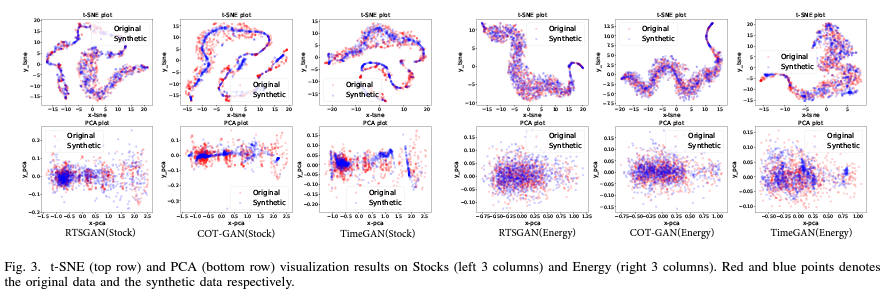B. Incomplete variable-length time series data

We then evaluate the variable-length time series data with missing data. The datasets are the PhysioNet Challenge Dataset (multivariate medical data from the ICU) and MIMIC-IIL (data from the Medical Information Mart in the intensive care unit). details are represented in TABLE II.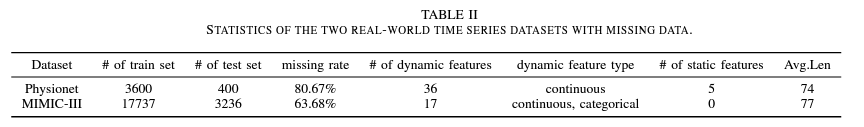The comparison targets are TimeGAN and DoppelGANger. The AUC comparison is performed after passing the downstream classification models zeroRNN and lastRNN. Scaling is done both min-max and standard. For qualitative evaluation, we use 2D graphical representation and Pearson correlation heatmap.

TABLE III and IV show the comparison results for the MIMIC-III and PhysioNet data, with RTSGAN and RTSGAN-M showing the best results. Fig. 4 shows the Pearson correlation heatmap for the MIMIC-III data, Fig. 5 shows a comparison of the t-SNE and PCA illustrations. Fig. 5 shows a comparison of the t-SNE and PCA illustrations, showing that RTSGAN's original distribution (red) is best reproduced by the generated data (blue).